Convex Lens - Image Formation and Magnification

# Our Objective

To study image formation by a convex lens using a lighted candle.

# The Theory

Lens: The lens is a transparent medium bounded by two surfaces one or both of which may be curved.

Convex Lens: A lens with both its sides bulging outward in the middle is a convex lens. It is also known as a converging lens. Light rays when refracted through a lens obeys the laws of refraction.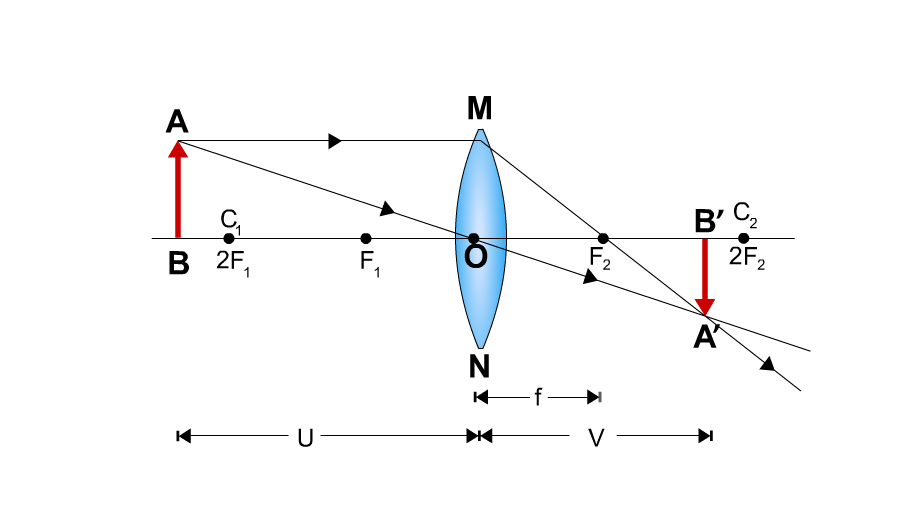• O = optical centre of the lens.
• F1, F2 = Foci of the lens. A lens has a focus on either side.
• 2F1, 2F2= Points that lie at a distance twice that of the focal length from O
• AB = object kept in front of the lens
• A’B’ = image of the object obtained on a screen
• f = focal length of the lens
• u = object distance
• v = image distance

The nature, position, and size of the image formed depend on the position of an object. It can be studied by keeping the object AB, the lens, and the image A’B’ all on the same straight line and observing the image for different object distances.

## Lens formula

For a spherical lens, the object distance u and image distance v can be related to focal length using the lens formula -

1∕ f = (1 ∕ v )- (1∕ u)

Knowing any of these 2 variables, we can find the third one. This enables us to find focal length of the given lens by when u and v are known. Similarly, we can predict where the image will be formed for a given object distance, if we know the focal length of the lens.

## Types of Images

• Real image: Image formed on the screen when the refracted or reflected light rays actually intersect with each other. The image obtained is always inverted with respect to the object.
• Virtual image: If the reflected or refracted rays do not actually meet at a point but appear to diverge from a point, then the image formed is virtual. It cannot be obtained on a screen. Such images are always erect with respect to the object.

## Sign Convention

• The object is placed on the left side of the lens such that incident rays fall on the left side of the lens
• All distances are measured from the optical centre of the lens. The optical centre is considered the origin of the Cartesian plane.
• X-axis passing through the origin is considered as the principal axis. All distances measured to the right of the origin along the positive x-axis are taken as positive.
• Distances measured to the left of the origin along the negative x-axis are taken as negative.
• Distances measured perpendicular to and above the principal axis along the positive y-axis are taken as positive.
• Distances measured perpendicular to and below the principal axis along the negative y-axis are taken as negative.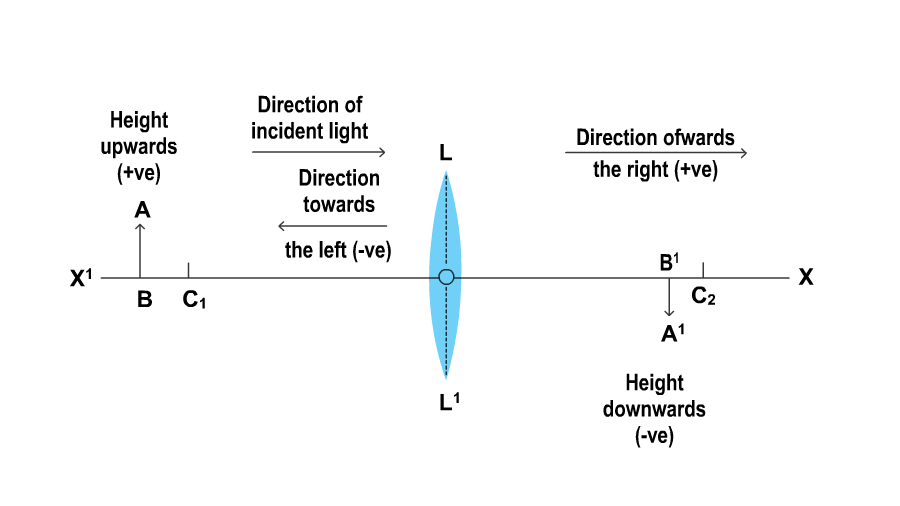The table summarises the formation of the image of an object formed by a thin convex lens when the object is placed at different positions

 Sl. No: Position of the object from the lens Position of the image from the lens Size of the image Nature of image 1. Beyond 2f Between f and 2f Diminished Real, inverted 2. At 2f At 2f Equal in size Real, inverted 3. Between f and 2f Beyond 2f Enlarged Real, inverted 4. At f At infinity Highly Enlarged Real, inverted 5. Between f and optical centre O On the same side of the lens Enlarged Virtual, Erect

Case 1:

When object is placed beyond 2f: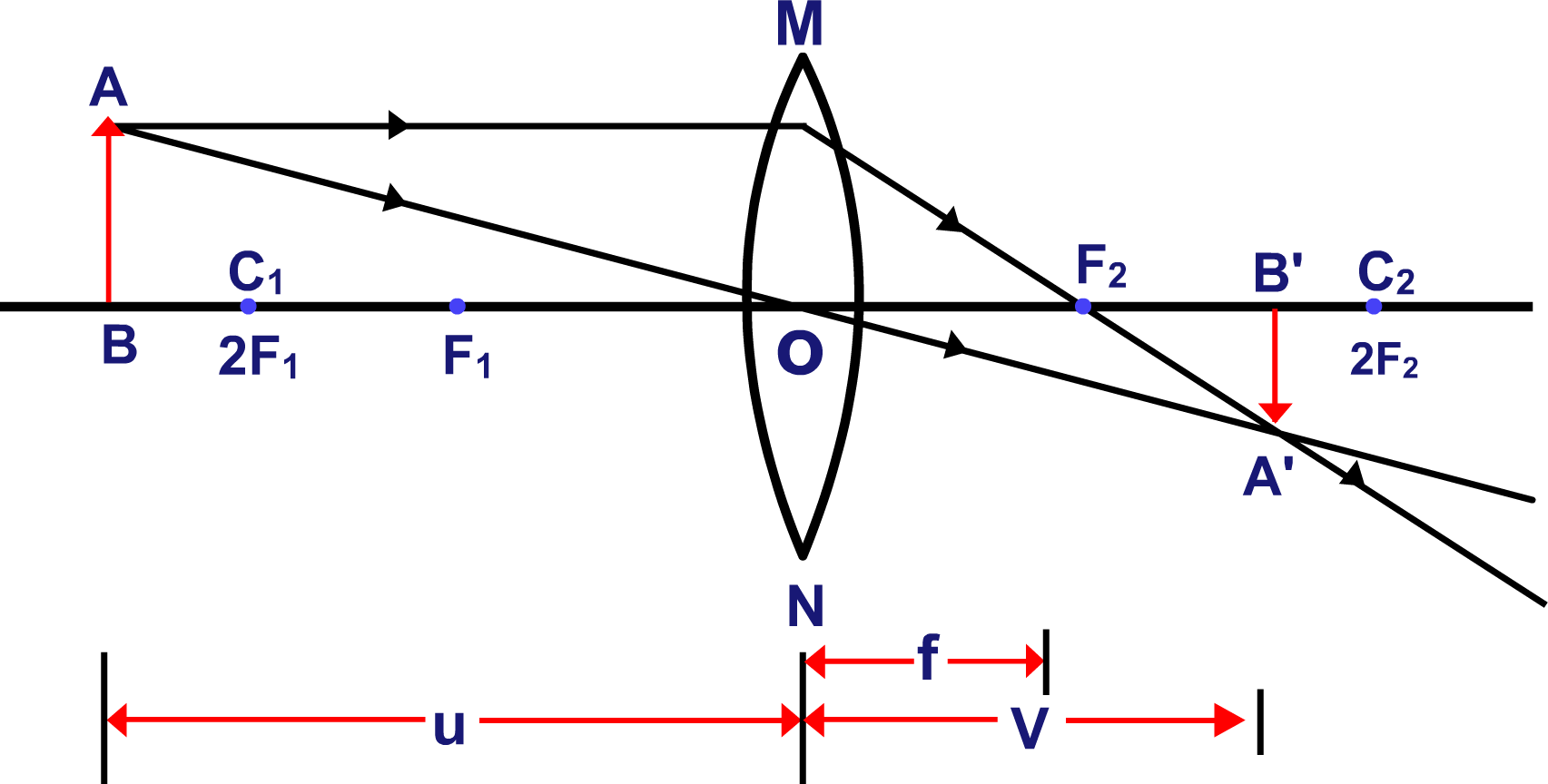Image is formed between F and 2F on the back side of lens

Case 2:

When object is placed at 2f: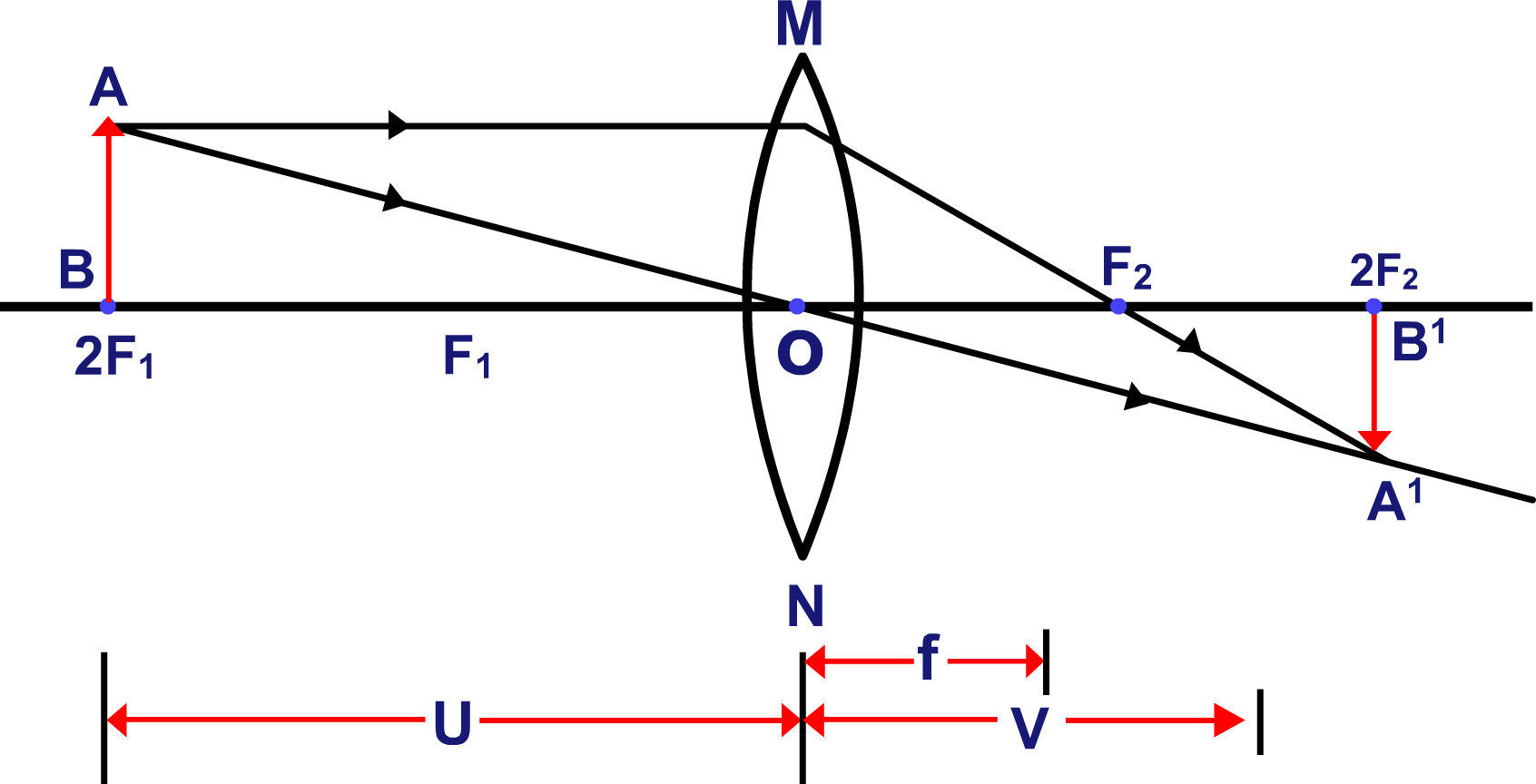Image is formed at 2F

Case 3:

When object is placed between 2f and f: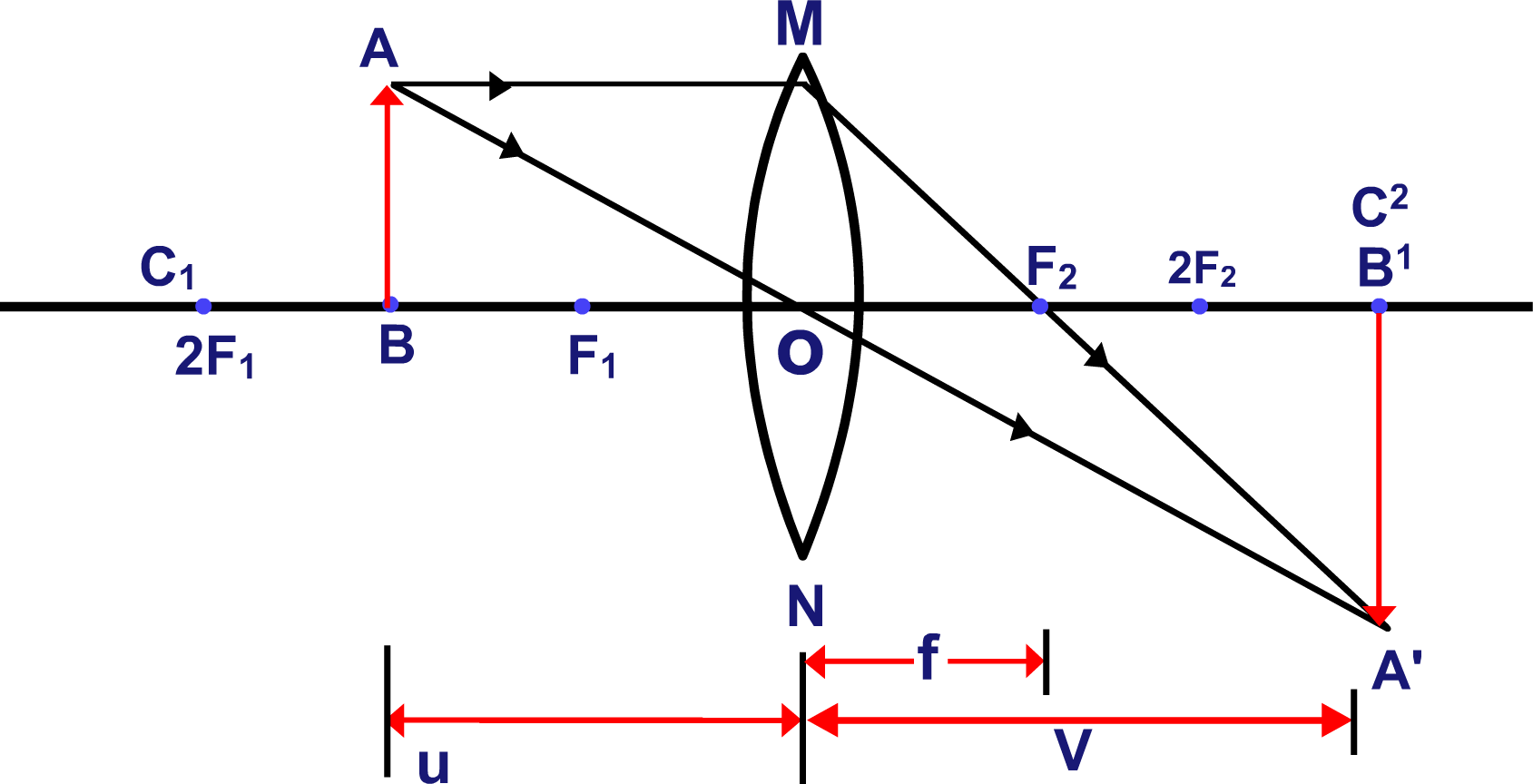Image is formed beyond 2f.

Case 4:

When object is placed at f: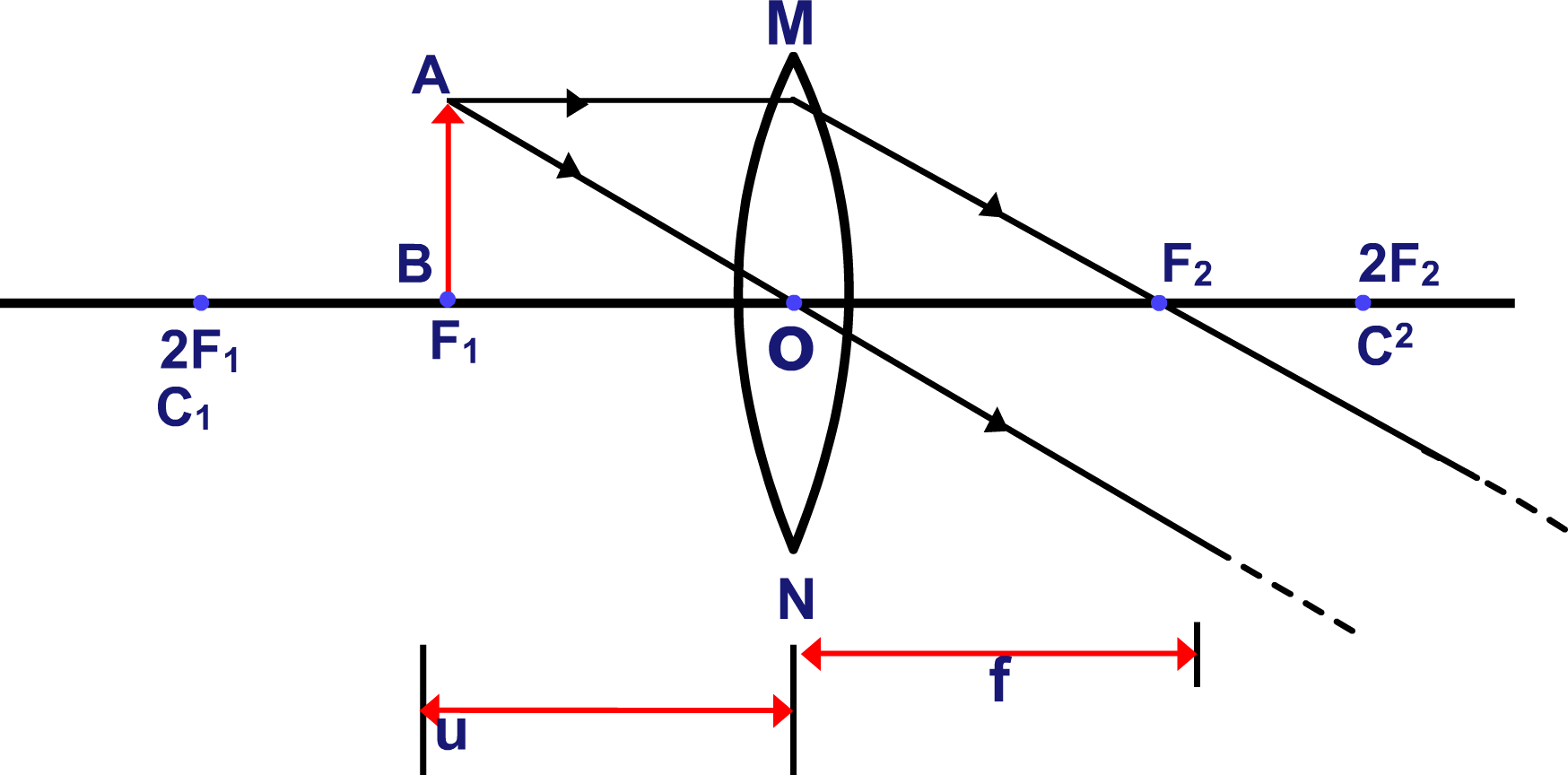Image is formed at infinity

Case 5:

When object is placed between focus and optical centre: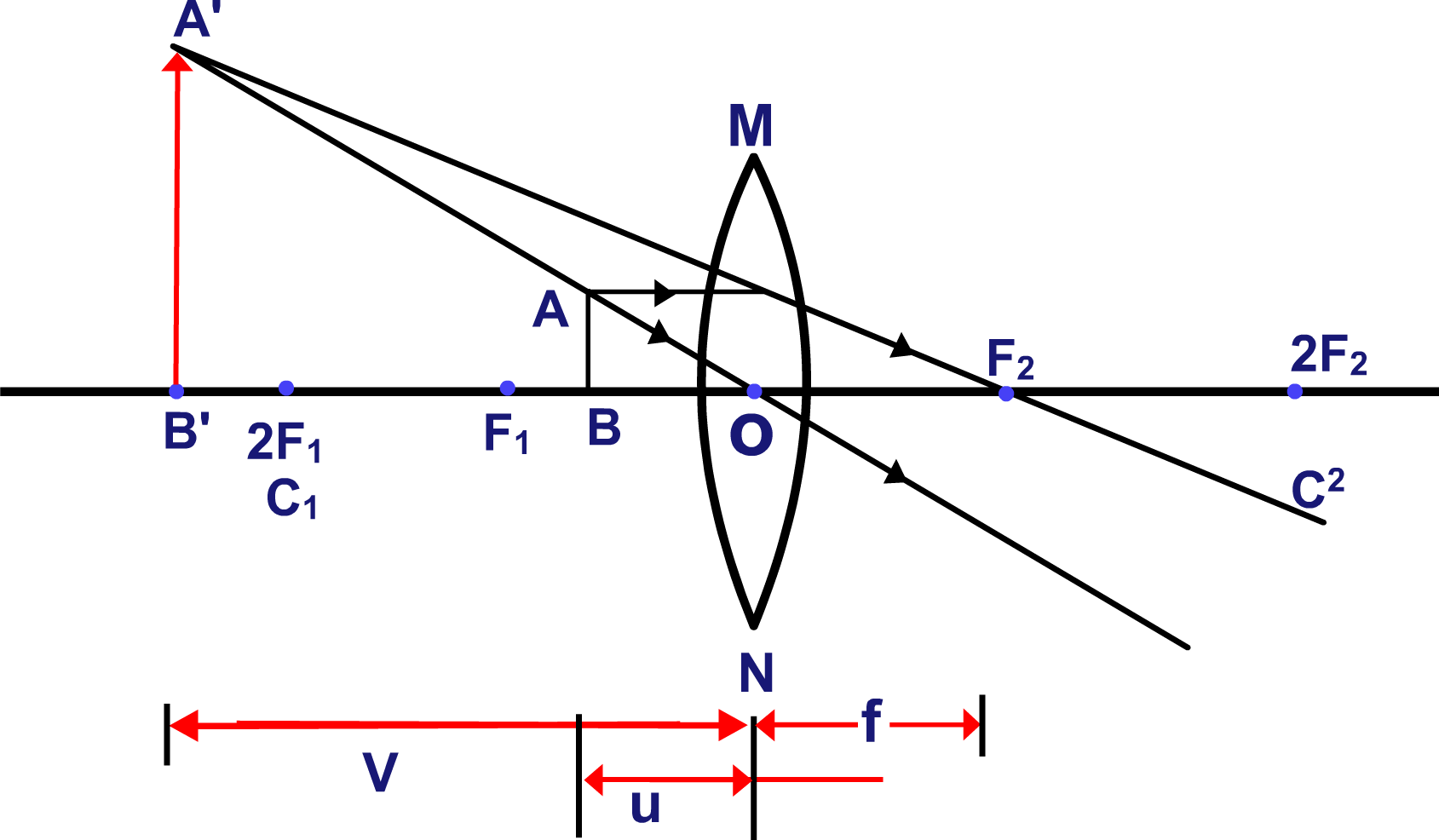Image is formed beyond 2F on the same side of object

## Magnification

Magnification produced by a lens is defined as the ratio of the height of the image (h’) to that of the object (h). It can also be related to the object distance (u) and the image distance (v).

M = h’/h = v/u

Magnification is negative for real and inverted images whereas it is positive for virtual and erect images.

# Learning Outcomes

• Nature, size, and position of the image with object distance for a convex lens are analysed.
• Students learn to apply the Lens formula.
• Students interpret the relation between magnification produced by a lens to the position of object and image.
• An understanding of the focal length and power of a converging lens is developed.
• Possible application of convex lens is inferred based on the image obtained.